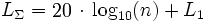Deutsche VersionTotal level adding of coherent signals
Fact: Voltage sum or Voltage addition

Coherent Signal Summing: This results from the fact that the sum voltage
will be twice the voltage of a single signal.

 Decibels are logarithmic "units" that can not be added to other numbers linearly. If a source creates a level L1 and a second source L2 that is built from the first, (as two closely powered loudspeakers get the same signal), then this gives a sum of the level which is 6 dB higher than the level of one signal. If both values are the same level, that's easy. The situation changes when both steady correlated or coherent combined levels are different. More than 6 dB higher than the higher of the two levels of the coherent sum level can never be reached. Note: This calculation here should not be confused with the usual addition of two acoustic "incoherent" (noncoherent) sources - such as a violin and a trumpet. See also the other case: ● Total level adding of acoustical incoherent (noncoherent) sound sources.

 Sum calculation of two coherent signals - "Electric level addition" 1st source  Level L1  dB |     |    |    | + 2nd source  Level L2  dB |     |    |    | Total level of   both sources    =  dB Coherent signals! "Voltage sum"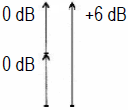Voltage sum coherent (0°) 1 + 1 = 2 Power sum incoherent (90°) √ (1² + 1²) = 1,414...

 Adding of two coherent pressure or voltage level: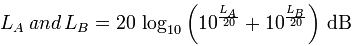Adding of two values of the same level results in an increase in the overall level here of (+) 6 dB. This is obtained with the equal input of two closely standing speakers.   Adding of two incoherent (noncoherent) pressure or voltage level: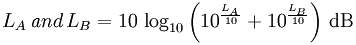Adding of two values of the same level results in an increase of the total level of (+) 3 dB. This equation is used for both the electric incoherent addition of signals, and for the calculation of the energy level of two loudspeakers.

Level addition of several coherent signals

 source 1 dB source 2 dB source 3 dB source 4 dB total sum dBAdding of equal strong coherent signals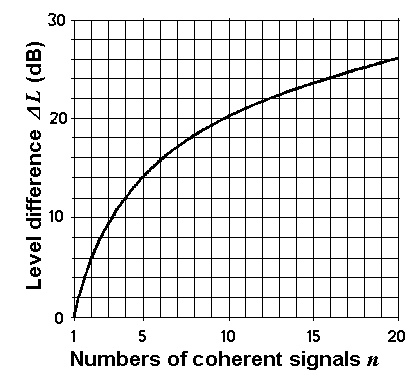Level increase Δ L for n equal coherent signals Number of n equal loud sound sources Level increase  Δ L in dB 1 0 2 6.0 3 9.6 4 12.0 5 14.0 6 15.6 7 17.0 8 18.0 9 19.0 10 20.0 12 21.6 16 24.0 20 26.0

Formulas: Δ L = 20 × log n  or  n = 10(ΔL/20)
Δ L = level difference; n = number of equal coherent sources.

 n = 2 coherent sources of equal level result in a higher level of 20 × log10 2 = +6.02 dB compared to the case that only one source is available. n = 3 coherent sources of equal level result in a higher level of 20 × log10 3 = +9.54 dB compared to the case that only one source is available. n = 4 coherent sources of equal level result in a higher level of 20 × log10 4 = +12.04 dB compared to the case that only one source is available. n = 10 coherent sources of equal level result in a higher level of 20 × log10 10 = +20.00 dB compared to the case that only one source is available.

Adding (combining) equal strong coherent sources

 Simply enter the value to the left or the right side. The calculator works in both directions of the ↔ sign.

 Adding equal strong coherent sources Number of sound sources n ↔ Increase of level Δ L dB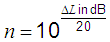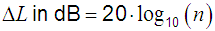The total level in dB is the level of one source plus the increase of level in dB.